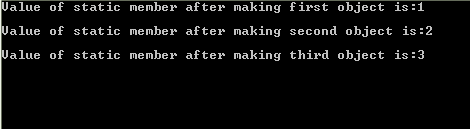# Static Data Members

In this tutorial you will learn: 1. What are Static Data Members? 2. Why to use Static Data Members? 3. How to use Static Data Members in program? 4. Basic C++ syntax What are Static Data Members? Static members are those data members that retain their value , whenever the static member is reused in the program. Static data members store the latest value in them so that the value will be used for next time when the function that makes use of static data member is called. The lifetime of the static data members is equal to the lifetime of the program. Static data members are initialized outside the class and usually initialized with the value zero. Why to use Static Data Members? In object oriented programming we have make objects of classes, suppose if we want to keep record of number of objects made by the user. So for the purpose we declare an integer type static data member and always increment by one when an object is formed. In this way we keep the record of the number of objects. Secondly another use is to keep the record of number of times when a certain function is called in a program. So whenever a specific function is called we increment the static data member by one hence it can give us count that how many times a function has been called. How to use Static Data Members? To make a Static Data member we use the keyword ‘static’ with any data type like float, int, double etc. It must be remembered here that static data members are initialized outside the class and are initialized to value zero. For Example: In this example we declared integer type static data member ‘staticNo’ Class someClass { Static int staticNo; //declaration } int someClass::staticNo=0; //initializing data member Program: strong>Static Data Member Basic Step: Open Dev C++ then File > new > source file and start writing the code below.
`#include<iostream>#include<conio.h>using namespace std;`
These are the basic header files which are required for C++ programming. Using namespace std is used at the top of the program because if its not written then we have to write ‘std::’ with every line of the program. #include includes the C++ built in libraries and iostream is used for input and output of the program. Conio.h is used to show output on the screen, if it is not present , the screen flashes and disappears hence not showing output.
`class myClass { private:	static int count; public:	myClass() 			{ count++;}	int getcount() 	{return count;}}; int myClass::count=0; `
In this program we have made a class named ‘myClass’ in which we have made only one data member that is also the static data member. We have made its default constructor in which we increment the count by 1 whenever the object is formed. Here count++ means count=count+1; In class we have made another function ‘getcount’, this function returns the value of one and only data member i.e count. The static data member count is initialized outside the class.
` void main()  { myClass obj1; cout<<"Value of static member after making first object is:"; cout<<obj1.getcount()<<"\n"; myClass obj2; cout<<"\nValue of static member after making second object is:"; cout<<obj2.getcount()<<"\n"; myClass obj3; cout<<"\nValue of static member after making third object is:"; cout<<obj3.getcount()<<"\n";  getch(); } `
In the main of the program we have made three objects of the class ‘myClass’ and the value of static member is displayed after the making of each object. After making each object the value of count is incremented by one hence the result after making third object the value of static member becomes three. Execute > compile then Execute > run Output